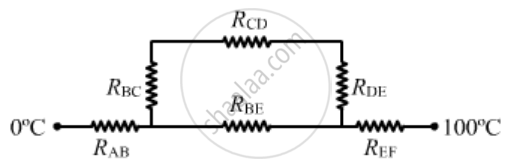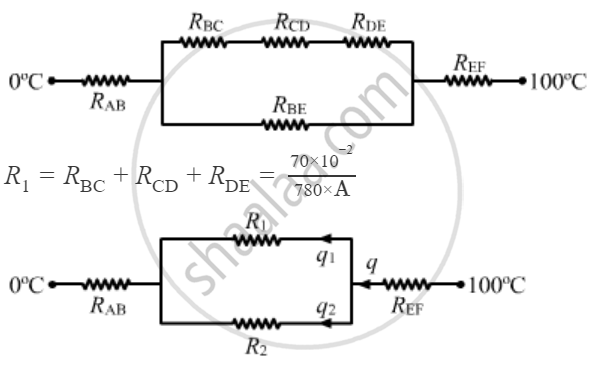Share

# Suppose the Bent Part of the Frame of the Previous Problem Has a Thermal Conductivity of 780 J S−1 M−1 °C−1 Whereas It is 390 J S−1 M−1°C−1 for the Straight Part. Calculate the Ratio of the Rate of - Physics

ConceptThermal Expansion of Solids

#### Question

Suppose the bent part of the frame of the previous problem has a thermal conductivity of 780 J s−1 m−1 °C−1 whereas it is 390 J s−1 m1°C−1 for the straight part. Calculate the ratio of the rate of heat flow through the bent part to the rate of heat flow through the straight part.

#### SolutionResistance of any branch, R = l/{KA}

Here, K is the thermal conductivity, A is the area of cross section and l is the length of the conductor.

R_{BC} = 1/{780.A} = {5xx10^-2}/{780.A}

R_{CD} = {60xx10^-2}/"780.A"

R_{DE} ={5xx10^-2}/{780.A}

R_{AB} = {20xx10^-2}/{390.A}

R_{EF} = {20xx10^-2}/{390.A}

R_{BE} = {60xx10^-2}/{390.A}R_(BE) = R_2 = (60xx10^-2)/(390xxA)

since R1 and R2 are in parallel, the amount of heat flowing through them will be same.

{q_1}/{q_2} = {R_2}/{R_1}

= {60xx10^-2xx780xxA}/{390xxAxx70xx10}

= 12/7

⇒ {q_1}/{q_2 }= 12/7

Is there an error in this question or solution?

#### APPEARS IN

Solution Suppose the Bent Part of the Frame of the Previous Problem Has a Thermal Conductivity of 780 J S−1 M−1 °C−1 Whereas It is 390 J S−1 M−1°C−1 for the Straight Part. Calculate the Ratio of the Rate of Concept: Thermal Expansion of Solids.
S